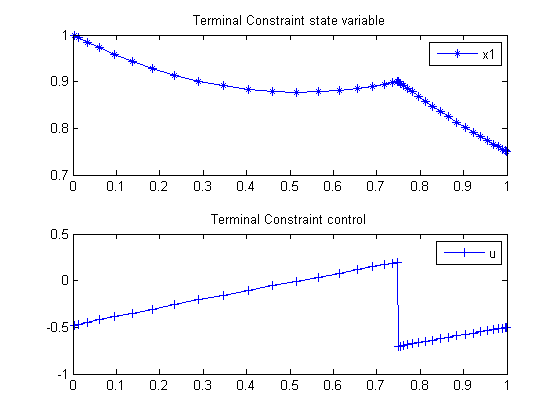111  A Simple Terminal Constraint Problem

Problem 1: Miser3 manual

111.1  Problem Description

Find u(t) over t in [0; 1 ] to minimize

J =
 1 0
(x2 + u2dt

subject to:

 dx dt
= u
 x(0) = 1
 x(1) = 0.75
 x(0.75) = 0.9

111.2  Problem setup

toms t1
p1 = tomPhase('p1', t1, 0, 0.75, 20);
toms t2
p2 = tomPhase('p2', t2, 0.75, 0.25, 20);

setPhase(p1);
tomStates x1p1
tomControls up1
setPhase(p2);
tomStates x1p2
tomControls up2
setPhase(p1);

% Initial guess
x01 = {icollocate({x1p1 == 1-0.1*t1/0.75})
collocate(up1==0.9*t1/0.75)};

% Box constraints
cbox1 = {-10 <= icollocate(p1,x1p1) <= 10
-10 <= collocate(p1,up1) <= 10};

% Boundary constraints
cbnd1 = initial(x1p1 == 1);

% ODEs and path constraints
ceq1 = collocate(dot(x1p1) == up1);

% Objective
objective1 = integrate(x1p1.^2+up1.^2);

setPhase(p2);
% Initial guess
x02 = {icollocate({x1p2 == 1-0.1*t2})
collocate(up2==0.9+0.1*t2)};

% Box constraints
cbox2 = {-10 <= icollocate(p2,x1p2) <= 10
-10 <= collocate(p2,up2) <= 10};

% Boundary constraints
cbnd2 = {initial(x1p2 == 0.9)
final(x1p2 == 0.75)};

% ODEs and path constraints
ceq2 = collocate(dot(x1p2) == up2);

% Objective
objective2 = integrate(x1p2.^2+up2.^2);

% Objective
objective = objective1 + objective2;

111.3  Solve the problem

options = struct;
options.name = 'Terminal Constraint 2';
constr = {cbox1, cbnd1, ceq1, cbox2, cbnd2, ceq2, link};
solution = ezsolve(objective, constr, {x01, x02}, options);

t = subs(collocate(p1,t1),solution);
t = [t;subs(collocate(p2,t2),solution)];
x1 = subs(collocate(p1,x1p1),solution);
x1 = [x1;subs(collocate(p2,x1p2),solution)];
u = subs(collocate(p1,up1),solution);
u = [u;subs(collocate(p2,up2),solution)];
Problem type appears to be: qp
Starting numeric solver
===== * * * =================================================================== * * *
TOMLAB - Tomlab Optimization Inc. Development license  999001. Valid to 2011-02-05
=====================================================================================
Problem:  1: Terminal Constraint 2              f_k       0.920531441472477340
sum(|constr|)      0.000000000559899399
f(x_k) + sum(|constr|)      0.920531442032376690
f(x_0)      0.000000000000000000

Solver: CPLEX.  EXIT=0.  INFORM=1.
CPLEX Barrier QP solver
Optimal solution found

FuncEv    8 GradEv    8 ConstrEv    8 Iter    8
CPU time: 0.031250 sec. Elapsed time: 0.031000 sec.

111.4  Plot result

subplot(2,1,1)
plot(t,x1,'*-');
legend('x1');
title('Terminal Constraint state variable');

subplot(2,1,2)
plot(t,u,'+-');
legend('u');
title('Terminal Constraint control');